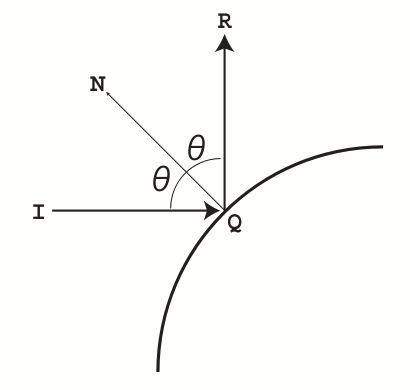Time Limit : sec, Memory Limit : KB
English

# Problem E: Spherical Mirrors

A long time ago in a galaxy, far, far away, there were N spheres with various radii. Spheres were mirrors, that is, they had reflective surfaces . . . .

You are standing at the origin of the galaxy (0, 0, 0), and emit a laser ray to the direction (u,v, w). The ray travels in a straight line.

When the laser ray from I hits the surface of a sphere at Q, let N be a point outside of the sphere on the line connecting the sphere center and Q. The reflected ray goes to the direction towards R that satisfies the following conditions: (1) R is on the plane formed by the three points I, Q and N , (2) ∠ IQN = ∠ NQR, as shown in Figure 1.

Figure 1: Laser ray reflectionAfter it is reflected several times, finally it goes beyond our observation. Your mission is to write a program that identifies the last reflection point.

## Input

The input consists of multiple datasets, each in the following format.

       N
u v w
x1 y1 z1 r1
.
.
.
xN yN zN rN


The first line of a dataset contains a positive integer N which is the number of spheres. The next line contains three integers u, v and w separated by single spaces, where (u, v, w) is the direction of the laser ray initially emitted from the origin.

Each of the following N lines contains four integers separated by single spaces. The i-th line corresponds to the i-th sphere, and the numbers represent the center position (xi, yi, zi ) and the radius ri .

N, u, v, w, xi, yi, zi and ri satisfy the following conditions.

1 ≤ N ≤ 100
−100 ≤ u, v, w ≤ 100
−100 ≤ xi, yi, zi ≤ 100
5 ≤ ri ≤ 30
u2 + v2 + w2 > 0

You can assume that the distance between the surfaces of any two spheres is no less than 0.1. You can also assume that the origin (0, 0, 0) is located outside of any sphere, and is at least 0.1 distant from the surface of any sphere.

The ray is known to be reflected by the sphere surfaces at least once, and at most five times. You can assume that the angle between the ray and the line connecting the sphere center and the reflection point, which is known as the angle of reflection (i.e. θ in Figure 1), is less than 85 degrees for each point of reflection.

The last dataset is followed by a line containing a single zero.

## Output

For each dataset in the input, you should print the x-, y- and z-coordinates of the last reflection point separated by single spaces in a line. No output line should contain extra characters.

No coordinate values in the output should have an error greater than 0.01.

## Sample Input

3
-20 -20 -24
100 100 100 30
10 8 3 5
-70 -70 -84 5
4
0 47 84
-23 41 42 8
45 -10 14 19
-5 28 47 12
-27 68 34 14
0


## Output for the Sample Input

79.0940 79.0940 94.9128
-21.8647 54.9770 34.1761